# Impedance

Single phase linear impedance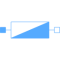# Information

This information is part of the Modelica Standard Library maintained by the Modelica Association.

The impedance model represents a series connection of a resistor and either an inductor or capacitor.The linear impedance connects the voltage `v` with the current `i` by `v = Z*i`. The resistive component is modeled temperature dependent, so the real part `R_actual = real(Z)` is determined from the actual operating temperature and the reference input resistance `real(Z_ref)`. A conditional heat port is considered. The reactive component `X_actual = imag(Z)` is equal to `imag(Z_ref)` if `frequencyDependent = false`. Frequency dependency is considered by `frequencyDependent = true`, distinguishing two cases:

(a) `imag(Z_ref) > 0`: inductive case
The actual reactance `X_actual` is proportional to `f/f_ref`
(b) `imag(Z_ref) < 0`: capacitive case
The actual reactance `X_actual` is proportional to `f_ref/f`

# Parameters (9)

useHeatPort Value: false Type: Boolean Description: =true, if heatPort is enabled Value: T_ref Type: Temperature (K) Description: Fixed device temperature if useHeatPort = false Value: Type: ComplexImpedance Description: Complex impedance R_ref + j*X_ref Value: 293.15 Type: Temperature (K) Description: Reference temperature Value: 0 Type: LinearTemperatureCoefficient (¹/K) Description: Temperature coefficient of resistance (R_actual = R_ref*(1 + alpha_ref*(heatPort.T - T_ref)) Value: false Type: Boolean Description: Consider frequency dependency, if true Value: 1 Type: Frequency (Hz) Description: Reference frequency, if frequency dependency is considered Value: real(Z_ref) Type: Resistance (Ω) Description: Resistive component of impedance, resistance Value: imag(Z_ref) Type: Reactance (Ω) Description: Reactive component of impedance, reactance

# Connectors (3)

pin_p pin_n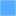Type: PositivePin Description: Positive quasi-static single-phase pin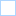Type: NegativePin Description: Negative quasi-static single-phase pin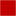Type: HeatPort_a Description: Conditional heat port

# Components (3)

v i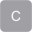Type: ComplexVoltage Description: Complex voltageType: ComplexCurrent Description: Complex currentType: ComplexImpedance Description: Complex impedance R_ref + j*X_ref

# Used in Examples (1)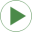Transformer Modelica.Electrical.QuasiStationary.SinglePhase.Examples Example of transformer with short circuit impedance, transmission resistances and load

# Used in Components (1)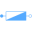Impedance Modelica.Electrical.QuasiStationary.MultiPhase.Basic Multiphase linear impedance